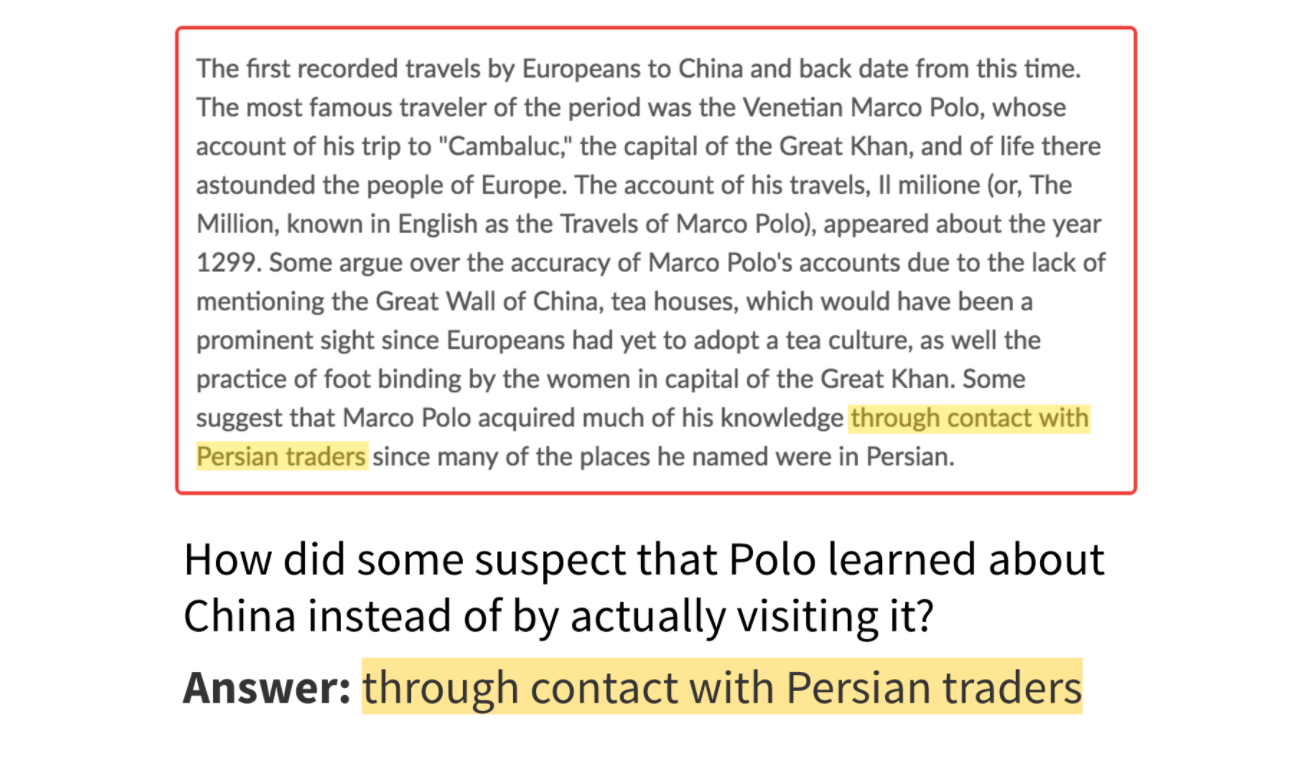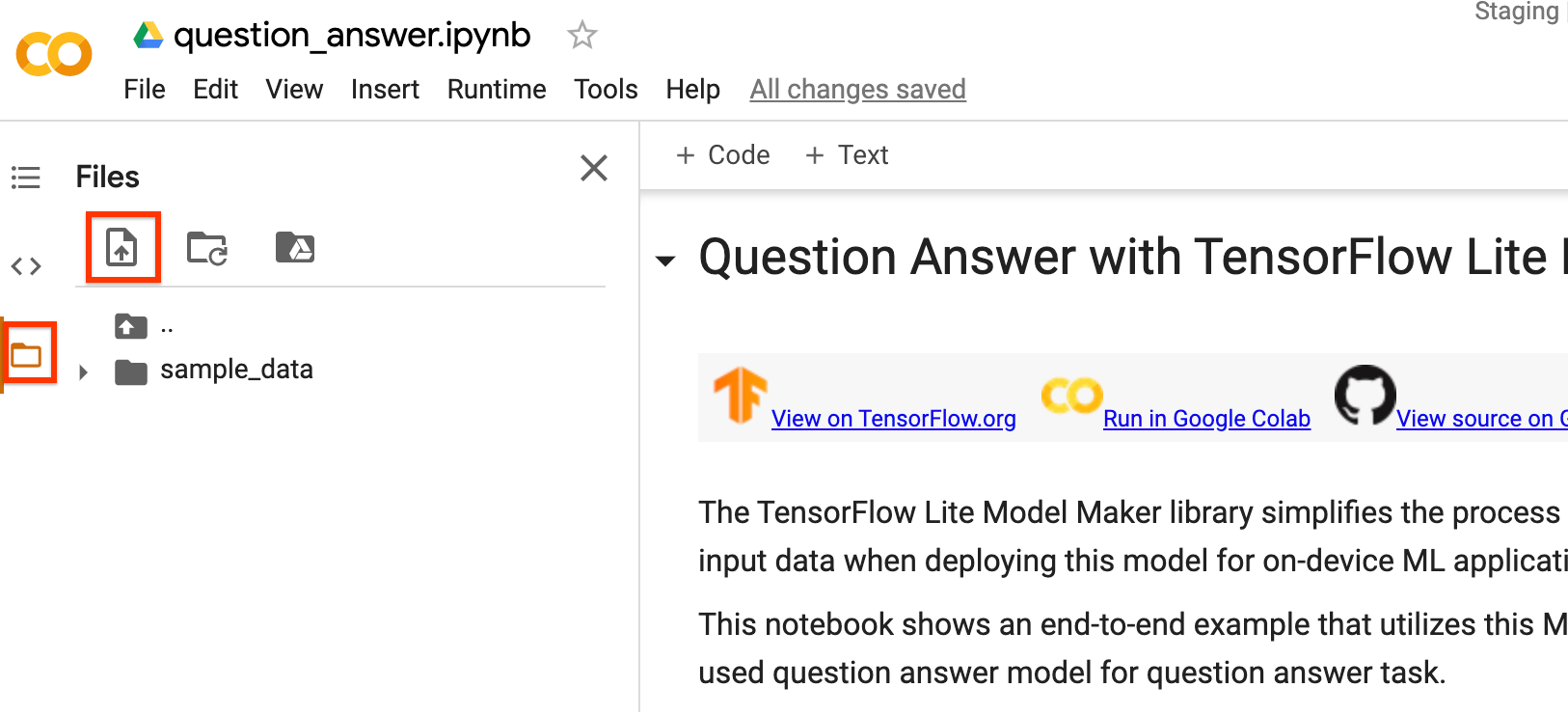Save the date! Google I/O returns May 18-20

# BERT Question Answer with TensorFlow Lite Model Maker

The TensorFlow Lite Model Maker library simplifies the process of adapting and converting a TensorFlow model to particular input data when deploying this model for on-device ML applications.

This notebook shows an end-to-end example that utilizes the Model Maker library to illustrate the adaptation and conversion of a commonly-used question answer model for question answer task.

# Introduction to BERT Question Answer Task

The supported task in this library is extractive question answer task, which means given a passage and a question, the answer is the span in the passage. The image below shows an example for question answer.Answers are spans in the passage (image credit: SQuAD blog)

As for the model of question answer task, the inputs should be the passage and question pair that are already preprocessed, the outputs should be the start logits and end logits for each token in the passage. The size of input could be set and adjusted according to the length of passage and question.

## End-to-End Overview

The following code snippet demonstrates how to get the model within a few lines of code. The overall process includes 5 steps: (1) choose a model, (2) load data, (3) retrain the model, (4) evaluate, and (5) export it to TensorFlow Lite format.

``````# Chooses a model specification that represents the model.
spec = model_spec.get('mobilebert_qa')

# Gets the training data and validation data.
train_data = QuestionAnswerDataLoader.from_squad(train_data_path, spec, is_training=True)
validation_data = QuestionAnswerDataLoader.from_squad(validation_data_path, spec, is_training=False)

# Fine-tunes the model.
model = question_answer.create(train_data, model_spec=spec)

# Gets the evaluation result.
metric = model.evaluate(validation_data)

# Exports the model to the TensorFlow Lite format with metadata in the export directory.
model.export(export_dir)
``````

The following sections explain the code in more detail.

## Prerequisites

To run this example, install the required packages, including the Model Maker package from the GitHub repo.

````pip install -q tflite-model-maker`
```

Import the required packages.

``````import numpy as np
import os

import tensorflow as tf
assert tf.__version__.startswith('2')

from tflite_model_maker import configs
from tflite_model_maker import ExportFormat
from tflite_model_maker import model_spec
from tflite_model_maker import question_answer
from tflite_model_maker import QuestionAnswerDataLoader
``````

The "End-to-End Overview" demonstrates a simple end-to-end example. The following sections walk through the example step by step to show more detail.

## Choose a model_spec that represents a model for question answer

Each `model_spec` object represents a specific model for question answer. The Model Maker currently supports MobileBERT and BERT-Base models.

Supported Model Name of model_spec Model Description
MobileBERT 'mobilebert_qa' 4.3x smaller and 5.5x faster than BERT-Base while achieving competitive results, suitable for on-device scenario.
MobileBERT-SQuAD 'mobilebert_qa_squad' Same model architecture as MobileBERT model and the initial model is already retrained on SQuAD1.1.
BERT-Base 'bert_qa' Standard BERT model that widely used in NLP tasks.

In this tutorial, MobileBERT-SQuAD is used as an example. Since the model is already retrained on SQuAD1.1, it could coverage faster for question answer task.

``````spec = model_spec.get('mobilebert_qa_squad')
``````

## Load Input Data Specific to an On-device ML App and Preprocess the Data

The TriviaQA is a reading comprehension dataset containing over 650K question-answer-evidence triples. In this tutorial, you will use a subset of this dataset to learn how to use the Model Maker library.

To load the data, convert the TriviaQA dataset to the SQuAD1.1 format by running the converter Python script with `--sample_size=8000` and a set of `web` data. Modify the conversion code a little bit by:

• Skipping the samples that couldn't find any answer in the context document;
• Getting the original answer in the context without uppercase or lowercase.

Download the archived version of the already converted dataset.

``````train_data_path = tf.keras.utils.get_file(
fname='triviaqa-web-train-8000.json',
origin='https://storage.googleapis.com/download.tensorflow.org/models/tflite/dataset/triviaqa-web-train-8000.json')
validation_data_path = tf.keras.utils.get_file(
fname='triviaqa-verified-web-dev.json',
origin='https://storage.googleapis.com/download.tensorflow.org/models/tflite/dataset/triviaqa-verified-web-dev.json')
``````
```Downloading data from https://storage.googleapis.com/download.tensorflow.org/models/tflite/dataset/triviaqa-web-train-8000.json
32571392/32570663 [==============================] - 0s 0us/step
Downloading data from https://storage.googleapis.com/download.tensorflow.org/models/tflite/dataset/triviaqa-verified-web-dev.json
1171456/1167744 [==============================] - 0s 0us/step
```

You can also train the MobileBERT model with your own dataset. If you are running this notebook on Colab, upload your data by using the left sidebar.If you prefer not to upload your data to the cloud, you can also run the library offline by following the guide.

Use the `QuestionAnswerDataLoader.from_squad` method to load and preprocess the SQuAD format data according to a specific `model_spec`. You can use either SQuAD2.0 or SQuAD1.1 formats. Setting parameter `version_2_with_negative` as `True` means the formats is SQuAD2.0. Otherwise, the format is SQuAD1.1. By default, `version_2_with_negative` is `False`.

``````train_data = QuestionAnswerDataLoader.from_squad(train_data_path, spec, is_training=True)
validation_data = QuestionAnswerDataLoader.from_squad(validation_data_path, spec, is_training=False)
``````

## Customize the TensorFlow Model

Create a custom question answer model based on the loaded data. The `create` function comprises the following steps:

1. Creates the model for question answer according to `model_spec`.
2. Train the question answer model. The default epochs and the default batch size are set according to two variables `default_training_epochs` and `default_batch_size` in the `model_spec` object.
``````model = question_answer.create(train_data, model_spec=spec)
``````
```INFO:tensorflow:Retraining the models...
INFO:tensorflow:Retraining the models...
Epoch 1/2
1067/1067 [==============================] - 405s 336ms/step - loss: 1.3041 - start_positions_loss: 1.3082 - end_positions_loss: 1.3000
Epoch 2/2
1067/1067 [==============================] - 359s 337ms/step - loss: 0.8425 - start_positions_loss: 0.8441 - end_positions_loss: 0.8408
```

Have a look at the detailed model structure.

``````model.summary()
``````
```Model: "model"
__________________________________________________________________________________________________
Layer (type)                    Output Shape         Param #     Connected to
==================================================================================================
input_word_ids (InputLayer)     [(None, 384)]        0
__________________________________________________________________________________________________
input_mask (InputLayer)         [(None, 384)]        0
__________________________________________________________________________________________________
input_type_ids (InputLayer)     [(None, 384)]        0
__________________________________________________________________________________________________
hub_keras_layer_v1v2 (HubKerasL {'end_logits': (None 24582914    input_word_ids
input_mask
input_type_ids
__________________________________________________________________________________________________
start_positions (Lambda)        (None, None)         0           hub_keras_layer_v1v2
__________________________________________________________________________________________________
end_positions (Lambda)          (None, None)         0           hub_keras_layer_v1v2
==================================================================================================
Total params: 24,582,914
Trainable params: 24,582,914
Non-trainable params: 0
__________________________________________________________________________________________________
```

## Evaluate the Customized Model

Evaluate the model on the validation data and get a dict of metrics including `f1` score and `exact match` etc. Note that metrics are different for SQuAD1.1 and SQuAD2.0.

``````model.evaluate(validation_data)
``````
```INFO:tensorflow:Made predictions for 200 records.
INFO:tensorflow:Made predictions for 200 records.
INFO:tensorflow:Made predictions for 400 records.
INFO:tensorflow:Made predictions for 400 records.
INFO:tensorflow:Made predictions for 600 records.
INFO:tensorflow:Made predictions for 600 records.
INFO:tensorflow:Made predictions for 800 records.
INFO:tensorflow:Made predictions for 800 records.
INFO:tensorflow:Made predictions for 1000 records.
INFO:tensorflow:Made predictions for 1000 records.
INFO:tensorflow:Made predictions for 1200 records.
INFO:tensorflow:Made predictions for 1200 records.
{'exact_match': 0.5918367346938775, 'final_f1': 0.6654577848455399}
```

## Export to TensorFlow Lite Model

Convert the existing model to TensorFlow Lite model format that you can later use in an on-device ML application.

Since MobileBERT is too big for on-device applications, use dynamic range quantization on the model to compress MobileBERT by 4x with the minimal loss of performance. First, define the quantization configuration:

``````config = configs.QuantizationConfig.create_dynamic_range_quantization(optimizations=[tf.lite.Optimize.OPTIMIZE_FOR_LATENCY])
config.experimental_new_quantizer = True
``````

Export the quantized TFLite model according to the quantization config with metadata. The default TFLite model filename is `model.tflite`.

``````model.export(export_dir='.', quantization_config=config)
``````
```INFO:tensorflow:Assets written to: /tmp/tmpyg0ktsxw/saved_model/assets
INFO:tensorflow:Assets written to: /tmp/tmpyg0ktsxw/saved_model/assets
INFO:tensorflow:Vocab file is inside the TFLite model with metadata.
INFO:tensorflow:Vocab file is inside the TFLite model with metadata.
INFO:tensorflow:Saved vocabulary in /tmp/tmpyhgyozge/vocab.txt.
INFO:tensorflow:Saved vocabulary in /tmp/tmpyhgyozge/vocab.txt.
Finished populating metadata and associated file to the model:
./model.tflite
The metadata json file has been saved to:
./model.json
The associated file that has been been packed to the model is:
['vocab.txt']
```

You can use the TensorFlow Lite model file in the bert_qa reference app using BertQuestionAnswerer API in TensorFlow Lite Task Library by downloading it from the left sidebar on Colab.

The allowed export formats can be one or a list of the following:

• `ExportFormat.TFLITE`
• `ExportFormat.VOCAB`
• `ExportFormat.SAVED_MODEL`

By default, it just exports TensorFlow Lite model with metadata. You can also selectively export different files. For instance, exporting only the vocab file as follows:

``````model.export(export_dir='.', export_format=ExportFormat.VOCAB)
``````
```INFO:tensorflow:Saved vocabulary in ./vocab.
INFO:tensorflow:Saved vocabulary in ./vocab.
```

You can also evaluate the tflite model with the `evaluate_tflite` method. This step is expected to take a long time.

``````model.evaluate_tflite('model.tflite', validation_data)
``````
```INFO:tensorflow:Made predictions for 100 records.
INFO:tensorflow:Made predictions for 100 records.
INFO:tensorflow:Made predictions for 200 records.
INFO:tensorflow:Made predictions for 200 records.
INFO:tensorflow:Made predictions for 300 records.
INFO:tensorflow:Made predictions for 300 records.
INFO:tensorflow:Made predictions for 400 records.
INFO:tensorflow:Made predictions for 400 records.
INFO:tensorflow:Made predictions for 500 records.
INFO:tensorflow:Made predictions for 500 records.
INFO:tensorflow:Made predictions for 600 records.
INFO:tensorflow:Made predictions for 600 records.
INFO:tensorflow:Made predictions for 700 records.
INFO:tensorflow:Made predictions for 700 records.
INFO:tensorflow:Made predictions for 800 records.
INFO:tensorflow:Made predictions for 800 records.
INFO:tensorflow:Made predictions for 900 records.
INFO:tensorflow:Made predictions for 900 records.
INFO:tensorflow:Made predictions for 1000 records.
INFO:tensorflow:Made predictions for 1000 records.
INFO:tensorflow:Made predictions for 1100 records.
INFO:tensorflow:Made predictions for 1100 records.
INFO:tensorflow:Made predictions for 1200 records.
INFO:tensorflow:Made predictions for 1200 records.
{'exact_match': 0.5850340136054422, 'final_f1': 0.6568895956651059}
```

## Advanced Usage

The `create` function is the critical part of this library in which the `model_spec` parameter defines the model specification. The `BertQAModelSpec` class is currently supported. There are 2 models: MobileBERT model, BERT-Base model. The `create` function comprises the following steps:

1. Creates the model for question answer according to `model_spec`.
2. Train the question answer model.

This section describes several advanced topics, including adjusting the model, tuning the training hyperparameters etc.

### Adjust the model

You can adjust the model infrastructure like parameters `seq_len` and `query_len` in the `BertQAModelSpec` class.

Adjustable parameters for model:

• `seq_len`: Length of the passage to feed into the model.
• `query_len`: Length of the question to feed into the model.
• `doc_stride`: The stride when doing a sliding window approach to take chunks of the documents.
• `initializer_range`: The stdev of the truncated_normal_initializer for initializing all weight matrices.
• `trainable`: Boolean, whether pre-trained layer is trainable.

Adjustable parameters for training pipeline:

• `model_dir`: The location of the model checkpoint files. If not set, temporary directory will be used.
• `dropout_rate`: The rate for dropout.
• `learning_rate`: The initial learning rate for Adam.
• `predict_batch_size`: Batch size for prediction.
• `tpu`: TPU address to connect to. Only used if using tpu.

For example, you can train the model with a longer sequence length. If you change the model, you must first construct a new `model_spec`.

``````new_spec = model_spec.get('mobilebert_qa')
new_spec.seq_len = 512
``````

The remaining steps are the same. Note that you must rerun both the `dataloader` and `create` parts as different model specs may have different preprocessing steps.

### Tune training hyperparameters

You can also tune the training hyperparameters like `epochs` and `batch_size` to impact the model performance. For instance,

• `epochs`: more epochs could achieve better performance, but may lead to overfitting.
• `batch_size`: number of samples to use in one training step.

For example, you can train with more epochs and with a bigger batch size like:

``````model = question_answer.create(train_data, model_spec=spec, epochs=5, batch_size=64)
``````

### Change the Model Architecture

You can change the base model your data trains on by changing the `model_spec`. For example, to change to the BERT-Base model, run:

``````spec = model_spec.get('bert_qa')
``````

The remaining steps are the same.